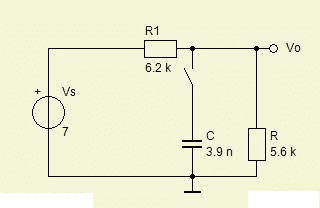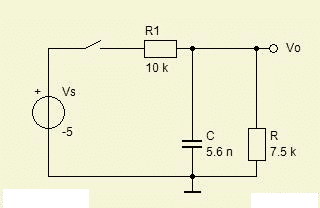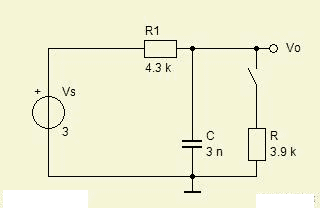# RC Circuit Transient Response

• Engineering
Homework Statement

In the circuit shown below the switch has been opened for a very long time and closes at
t = 0. Calculate the output voltage VO at:
1: t = 0−; 2: t = 0+; 3: t = t1 = 24 µs; 4: t → ∞.In the circuit shown below the switch has been closed for a very long time and opens at
t = 0. Calculate the output voltage VO at:
1: t = 0−; 2: t = 0+; 3: t = t1 = 24 µs; 4: t → ∞.The attempt at a solution

The only part I have trouble on is the getting the output voltage at t1 because I don't know how to obtain the equation in order to get the answer.

## Answers and Replies

gneill
Mentor
The only part I have trouble on is the getting the output voltage at t1 because I don't know how to obtain the equation in order to get the answer.

You need to determine the time constant for the circuit and write the expression for the voltage as a function of time. Replacing the resistor network and voltage source with a Thevenin equivalent may help with that.

I calculated Vo.e^(-t/RC) using the advice you gave but I got the wrong answer

gneill
Mentor
I calculated Vo.e^(-t/RC) using the advice you gave but I got the wrong answer

You don't provide enough information to see where you went wrong; You'll have to post your calculations.

for a question similar to the last one only but t1=3.6µs the correct answer is -11.2066V. I calculated the equivalent resistance of the two parallel resistors being 750 ohms. Therefore
RC = 2.25µs and -t/RC = -1.6 and finally using V0.e^(-t/RC) I get -1.6404V which is incorrect.

gneill
Mentor
for a question similar to the last one only but t1=3.6µs the correct answer is -11.2066V. I calculated the equivalent resistance of the two parallel resistors being 750 ohms. Therefore
RC = 2.25µs and -t/RC = -1.6 and finally using V0.e^(-t/RC) I get -1.6404V which is incorrect.

This doesn't help! I can't see the 'similar question'. Besides, you've got three different circuits in this thread. How are we to know which circuit your similar problem resembles?

Why don't you take one of your circuits that you're having problems with and detail your attempt at solution? Working though one may make the others clear.

Sorry about that, the 'similar question' is:

In the circuit shown below the switch has been closed for a very long time and opens at
t = 0. Calculate the output voltage VO at:
1: t = 0−; 2: t = 0+; 3: t = t1 = 3.6 µs; 4: t → ∞.

View attachment 39461

which the answer is -11.2066V for t1.

gneill
Mentor
Sorry about that, the 'similar question' is:

In the circuit shown below the switch has been closed for a very long time and opens at
t = 0. Calculate the output voltage VO at:
1: t = 0−; 2: t = 0+; 3: t = t1 = 3.6 µs; 4: t → ∞.

View attachment 39461

which the answer is -11.2066V for t1.

How is that possible? The for the given circuit the voltage source is only 3V. When the switch opens the output can only rise to that.

Oh sorry there must be something wrong it should read -13V for the voltage source and 1200 for R1 and 2000 for R

gneill
Mentor
Oh sorry there must be something wrong it should read -13V for the voltage source and 1200 for R1 and 2000 for R

Okay. Let's take those values. What value do you calculate for the output voltage before the switch is opened?

i get -13*(2000/3200) = -8.125V

gneill
Mentor
i get -13*(2000/3200) = -8.125V

Okay, that looks good. Now, what happens when the switch is opened? What is the initial value of Vo, and where will it head?

It will be the same.

gneill
Mentor
It will be the same.

and.... where will it head? What value will it decay towards as time goes on?

It will head to -13V I think.

gneill
Mentor
It will head to -13V I think.

Yes, that's right. So it will start at -8.125V and head to -13V. What's the time constant?

RC = 750*3n

gneill
Mentor
RC = 750*3n

Okay, looks good. So how would you write an equation to describe the voltage transitioning from -8.125V to -13V with that time constant?

EDIT: My bad. When the switch opens, only R1 = 1200Ω is in series with the capacitor. So RC is 1200Ω * 3nF.

Last edited:
Vo*e^(-t/RC) = -8.125e^(-3.6/2.25)

gneill
Mentor
Vo*e^(-t/RC) = -8.125e^(-3.6/2.25)

Vo is the output voltage. It's what the equation should be describing. I think you meant to use another variable name there. Let's call it Vth, which will have the value Vth = -8.125. So then you've provided:
$$Vo(t) = V_{th} e^{-\frac{t}{RC}}$$
This equation would describe an exponential decay from -8.125V up to zero volts. Not quite what you want. You should be ending at -13V as time goes on.

The form you want is:
$$Vo(t) = V_{start} + \Delta V \left(1 - e^{-\frac{t}{RC}}\right)$$
where $\Delta V = V_{end} - V_{start}$

This will describe a voltage curve that begins with Vstart and decays to Vend. (Plug in Vth and Vs for Vstart and Vend)

I got -12.016V instead of the correct answer -11.2066V.

gneill
Mentor
I got -12.016V instead of the correct answer -11.2066V.

You'll have to show more of your calculations. Mine show the expected result. Perhaps you should check your time constant value.

I got -8.125-[(-13+8.125)*(1-e^(-3.6/2.25))] = -12.016

gneill
Mentor
I got -8.125-[(-13+8.125)*(1-e^(-3.6/2.25))] = -12.016

Okay, my fault. I didn't pay close enough attention to your time constant calculation above. When the switch is open, only R1 is in the circuit. So the time constant should involve only R1 and the capacitor. Sorry about that. Try again with the new value.

ok I got the answer but is the equation the same for the question shown below:

In the circuits shown below the switch has been opened for a very long time and closes at
t = 0. Calculate the output voltage VO at:
1: t = 0−; 2: t = 0+; 3: t = t1 = 5.6 µs; 4: t → ∞.
View attachment 39459 View attachment 39460